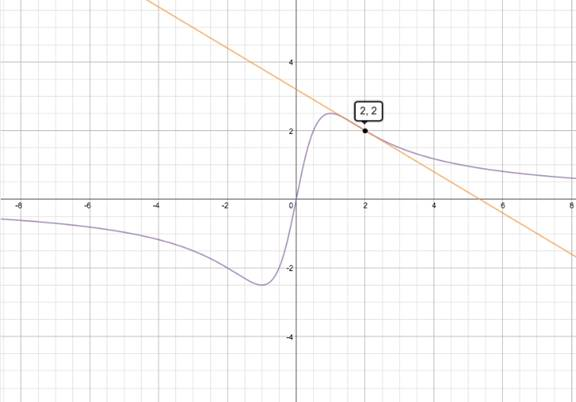# The equation of the tangent line to the curve y = 5 x / 1 + x 2 at the point ( 2 , 2 ) .### Single Variable Calculus: Concepts...

4th Edition
James Stewart
Publisher: Cengage Learning
ISBN: 9781337687805### Single Variable Calculus: Concepts...

4th Edition
James Stewart
Publisher: Cengage Learning
ISBN: 9781337687805

#### Solutions

Chapter 2.6, Problem 25E

a.

To determine

## To find:The equation of the tangent line to the curve y=5x/1+x2 at the point (2,2) .

Expert Solution

The tangent line is y=35x+165 and F'(2)=1525

### Explanation of Solution

Given: y=5x/1+x2 at the point (2,2) .

Concept used: g'(a)=limxa[g(x)g(a)]xa

Let y=5x/1+x2 be any function.

It has to find the tangent line of the curve and derivative of the function at a=2

g'(a)=limxa[g(x)g(a)]xa

F'(x)=5(1+x2)5x(2x)(1+x2)2F'(x)=55x2(1+x2)2F'(2)=55.22(1+22)2=1525

Point of interception is (2,2) plug in 2 for y1 and x1 solve the equation.

y(y1)=m(xx1)y2=35(x2)y=35(x2)+2y=35x+65+105y=35x+165

Hence, the tangent line is y=35x+165 and F'(2)=1525 .

b.

To determine

Expert Solution

### Explanation of Solution

Given: y=5x/1+x2 at the point (2,2) .

Let y=5x/1+x2 be any function.

In the graph

The purple curve is f(x)=5x1+x2

The orange line is the tangent at (2,2) .### Have a homework question?

Subscribe to bartleby learn! Ask subject matter experts 30 homework questions each month. Plus, you’ll have access to millions of step-by-step textbook answers!